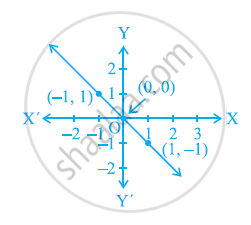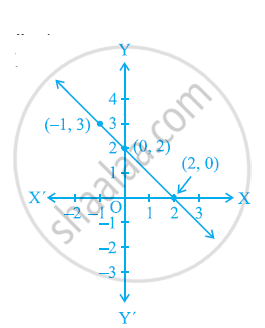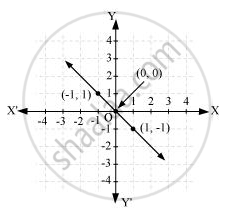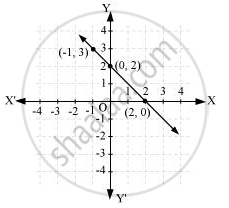# From the choices given below, choose the equation whose graphs are given in the given figures. - Mathematics

From the choices given below, choose the equation whose graphs are given in the given figures.

For the first figure

(i) y = x

(ii) x y = 0

(iii) y = 2x

(iv) 2 + 3y = 7xFor the second figure

(i) y = x +2

(ii) y = x − 2

(iii) y = − x + 2

(iv) x + 2y = 6#### SolutionPoints on the given line are (−1, 1), (0, 0), and (1, −1).

It can be observed that the coordinates of the points of the graph satisfy the equation x + y = 0. Therefore, x + y = 0 is the equation corresponding to the graph as shown in the first figure.

Hence, (ii) is the correct answer.Points on the given line are (−1, 3), (0, 2), and (2, 0). It can be observed that the coordinates of the points of the graph satisfy the equation y = − x + 2.

Therefore, = − x + 2 is the equation corresponding to the graph shown in the second figure.

Hence, (iii) is the correct answer.

Concept: Graph of a Linear Equation in Two Variables
Is there an error in this question or solution?

#### APPEARS IN

NCERT Class 9 Maths
Chapter 4 Linear Equations in two Variables
Exercise 4.3 | Q 5 | Page 74